# MP Board Class 10th Maths Solutions Chapter 6 Triangles Ex 6.4

In this article, we will share MP Board Class 10th Maths Book Solutions Chapter 6 Triangles Ex 6.4 Pdf, These solutions are solved subject experts from the latest edition books.

## MP Board Class 10th Maths Solutions Chapter 6 Triangles Ex 6.4

Question 1.
Let ∆ABC ~ ∆DEF and their areas be, respectively, 64 cm2 and 121 cm2. If EF = 15.4 cm, find BC.
Solution:
We have ar(∆ABC) = 64 cm2
ar(∆DEF) = 121 cm2 and EF = 15.4 cm[Given]
∵ ∆ABC ~ ∆DEF
∴ $$\frac { { ar }(\Delta ABC) }{ { ar }(\Delta DEF) } =\left( \frac { BC }{ EF } \right) ^{ 2 }$$
[Ratios of areas of two similar triangles is equal to the ratio of the squares of their corresponding sides]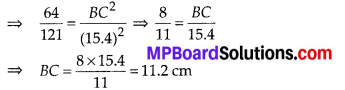Thus, BC = 11.2 cm

Question 2.
Diagonals of a trapezium ABCD with AB || DC intersect each other at the point O. If AB = 2CD, find the ratio of the areas of triangles AOB and COD.
Solution:
In trapezium ABCD, AB || DC. Diagonals AC and BD intersect at O.
In ∆AOB and ∆COD,∠AOB = ∠COD [Vertically opposite angles]
∠OAB = ∠OCD [Alternate angles]
∴ Using AA criterion of similarity, we have
∆AOB ~ ∆COD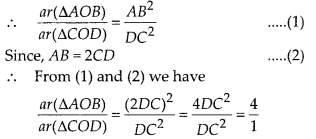i. e., ar(∆AOB) : ar(∆COD) = 4 : 1Question 3.
In the figure, ABC and DBC are two triangles on the same base BC. If AD intersects BC at O, show that $$\frac { { ar }(\Delta ABC) }{ { ar }(\Delta DBC) } =\frac { AO }{ DO }$$Solution:
We have, ∆ABC and ∆DBC are on the same base BC. Also BC and AD intersect at O.
Let us draw AE⊥BC and DF⊥BC.
In ∆AOE and ∆DOF,
∠AEO = ∠DFO = 90° ……….. (1)
Also, ∠AOE = ∠DOF …………… (2)
[Vertically Opposite Angles]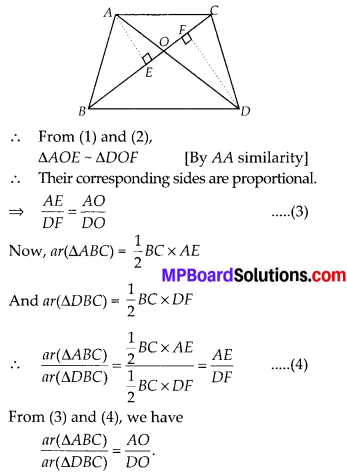Question 4.
If the areas of two similar triangles are equal, prove that they are congruent.
Solution:
We have ∆ABC and ∆DEF, such that ∆ABC ~ ∆DEF and ar(∆ABC) = ar(∆DEF).
Since the ratio of areas of two similar triangles is equal to the square of the ratio of their corresponding sides.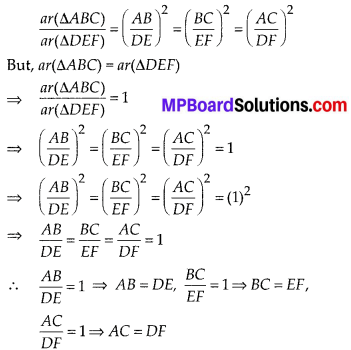i.e., the corresponding sides of ∆ABC and ∆DEF are equal.
⇒ ∆ABC ≅ ∆DEF [By SSS congruency]

Question 5.
D, E and F are respectively the mid-points of sides AB, BC and CA of ∆ABC. Find the ratio of the areas of ∆DEF and ∆ABC.
Solution:
We have a ∆ABC in which D, E and F are mid points of AB, BC and CA respectively. D, E and F are joined to form ∆DEF.Now, in ∆ABC, D and F are the mid-points of sides AB and AC.
∴ $$\frac{A D}{D B}=\frac{A F}{F C}$$ = 1
∴ By the converse of the basic proportion¬ality theorem, we have,
DF||BC ⇒ DF||BE
Similarly; EF||AB ⇒ EF||BD
Since, DF||BE and DB||EF
∴ Quadrilateral BEFD is a parallelogram.
⇒ FE = BD = $$\frac{1}{2}$$AB …………. (1)
Similarly, quadrilateral ECFD is a parallelogram.
⇒ DF = EC = $$\frac{1}{2}$$BC ………… (2)
and DE = FC = $$\frac{1}{2}$$AC ………….. (3)
Now, in ∆ABC and ∆DEF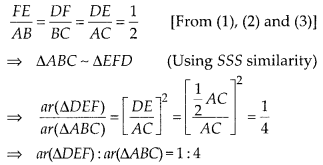Question 6.
Prove that the ratio of the areas of two similar triangles is equal to the square of the ratio of their corresponding medians.
Solution:
We have two triangles ABC and DEF such that ∆ABC ~ ∆DEFAM and DN are medians corresponding to BC and EF respectively.
∵ ∆ABC ~ ∆DEF
∴ The ratio of their areas is equal to the square of the ratio of their corresponding sides.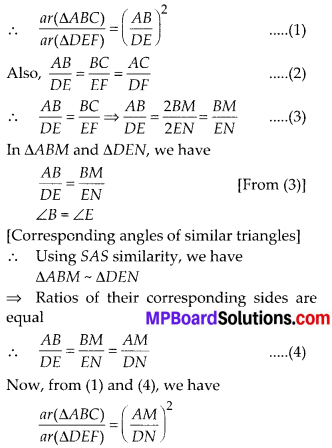Question 7.
Prove that the area of an equilateral triangle described on one side of a square is equal to half the area of the equilateral triangle described on one of its diagonals.
Solution:
We have a square ABCD, whose diagonal is AC. Equilateral ∆BQC is described on the side BC and another equilateral ∆APC is described on the diagonal AC.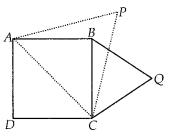∵ All equilateral triangles are similar.
∴ ∆APC ~ ∆BQC
∴ The ratio of their areas is equal to the square of the ratio of their corresponding sides.Tick the correct answer and justify:
Question 8.
ABC and BDE are two equilateral triangles such that D is the mid-point of BC. Ratio of the areas of triangles ABC and BDE is
(A) 2 : 1
(B) 1 : 2
(C) 4 : 1
(D) 1 : 4
Solution:
(C) : We have an equilateral ∆ABC and D is the mid point of BC. DE is drawn such that BDE is also an equilateral traingle. Since all equilateral triangles are similar,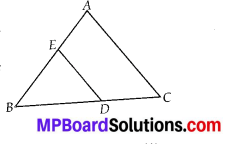∴ ∆ABC ~ ∆BDE
⇒ The ratio of their areas is equal to the square of the ratio of their corresponding sides.
∴ $$\frac { { ar }(\Delta ABC) }{ { ar }(\Delta BDE) } =\left( \frac { AB }{ BD } \right) ^{ 2 }$$ …………. (1)
∵ AB = AC = BCfsides of equilateral AABC]
and BD = $$\frac{1}{2}$$BC [∵ D is the mid point of BC]
⇒ BC = 2BD = AB …………… (2) [∵ AB = BC]
From (1) and (2), we have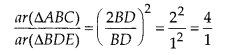⇒ ar(∆ABC) : ar(∆BDE) = 4 : 1

Question 9.
Sides of two similar triangles are in the ratio 4:9. Areas of these triangles are in the ratio
(A) 2 : 3
(B) 4 : 9
(C) 81 : 16
(D) 16 : 81
Solution:
(D) : We have two similar triangles such that the ratio of their corresponding sides is 4 : 9.
∴ The ratio of areas of two similar triangles is equal to the square of the ratio of their corresponding sides.
∴ $$\frac { { ar }(\Delta -I) }{ { ar }(\Delta -II) } =\left( \frac { 4 }{ 9 } \right) ^{ 2 }=\frac { 16 }{ 81 }$$
⇒ ar(∆-I): ar(∆-II) = 16 : 81